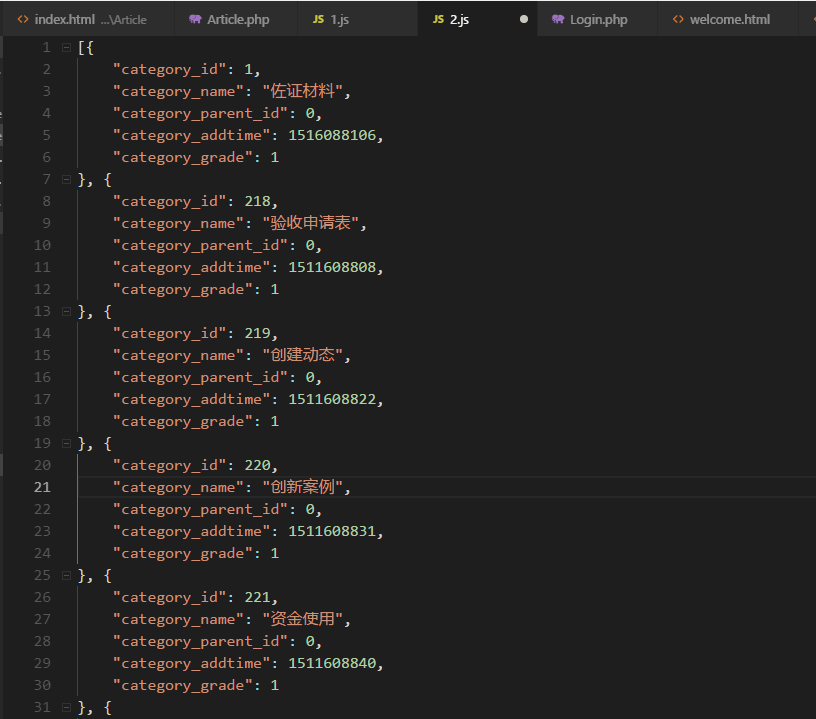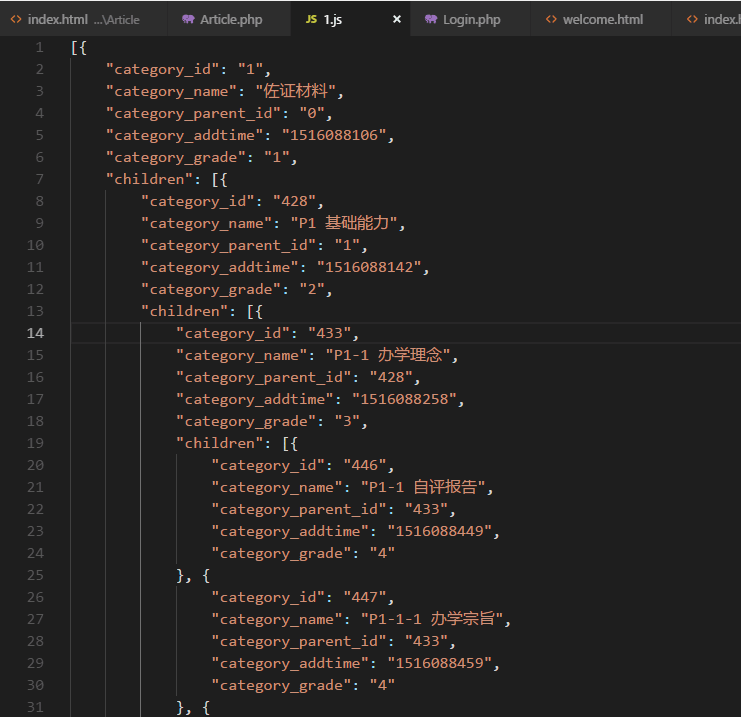thinkphp5框架实现数据库读取的数据转换成json格式示例

更新时间：2019年10月10日 10:41:22   作者：yangliwei.top:88我要评论

\$category = DB::name('Category');
\$category_list = \$category->select();
\$data=\$this->list_to_tree(\$category_list,'category_id','category_parent_id','children'); //调用下面的方法
\$data=\$this->findChild(\$data);
exit(json_encode(\$data,JSON_UNESCAPED_UNICODE));
// return \$this->fetch('index');
\$this->ajaxReturn(\$data, 'JSON');

function findChild(\$arr){
static \$tree=array();
foreach (\$arr as \$key=>\$val){
\$tree[]=\$val;
if (isset(\$val['_child'])){
\$this->findChild(\$val['_child']);
}
}
return \$tree;
}
/**
* 把返回的数据集转换成Tree
* @access public
* @param array \$list 要转换的数据集
* @param string \$pid parent标记字段
* @param string \$level level标记字段
* @return array
*/
function list_to_tree(\$list, \$pk='id',\$pid = 'pid',\$child = '_child',\$root=0) {
// 创建Tree
\$tree = array();
if(is_array(\$list)) {
// 创建基于主键的数组引用
\$refer = array();
foreach (\$list as \$key => \$data) {
\$refer[\$data[\$pk]] =& \$list[\$key];
}
foreach (\$list as \$key => \$data) {
// 判断是否存在parent
\$parentId = \$data[\$pid];
if (\$root == \$parentId) {
\$tree[] =& \$list[\$key];
}else{
if (isset(\$refer[\$parentId])) {
\$parent =& \$refer[\$parentId];
\$parent[\$child][] =& \$list[\$key];
}
}
}
}
return \$tree;
}PS：这里再为大家推荐几款比较实用的json在线工具供大家参考使用：

http://tools.jb51.net/code/json

JSON在线格式化工具：
http://tools.jb51.net/code/jsonformat

http://tools.jb51.net/code/xmljson

json代码在线格式化/美化/压缩/编辑/转换工具：
http://tools.jb51.net/code/jsoncodeformat